# Python | Customer Churn Analysis Prediction

Customer Churn
It is when an existing customer, user, subscriber, or any kind of return client stops doing business or ends the relationship with a company.

Types of Customer Churn –

• Contractual Churn : When a customer is under a contract for a service and decides to cancel the service e.g. Cable TV, SaaS.
• Voluntary Churn : When a user voluntarily cancels a service e.g. Cellular connection.
• Non-Contractual Churn : When a customer is not under a contract for a service and decides to cancel the service e.g. Consumer Loyalty in retail stores.
• Involuntary Churn : When a churn occurs without any request of the customer e.g. Credit card expiration.

Reasons for Voluntary Churn

• Lack of usage
• Poor service
• Better price

Code: Importing Telco Churn dataset

 `# Import required libraries ` `import` `numpy as np ` `import` `pandas as pd ` ` `  `# Import the dataset ` `dataset ``=` `pd.read_csv(``'telcochurndata.csv'``) ` ` `  `# Glance at the first five records ` `dataset.head() ` ` `  `# Print all the features of the data ` `dataset.columns `

Output: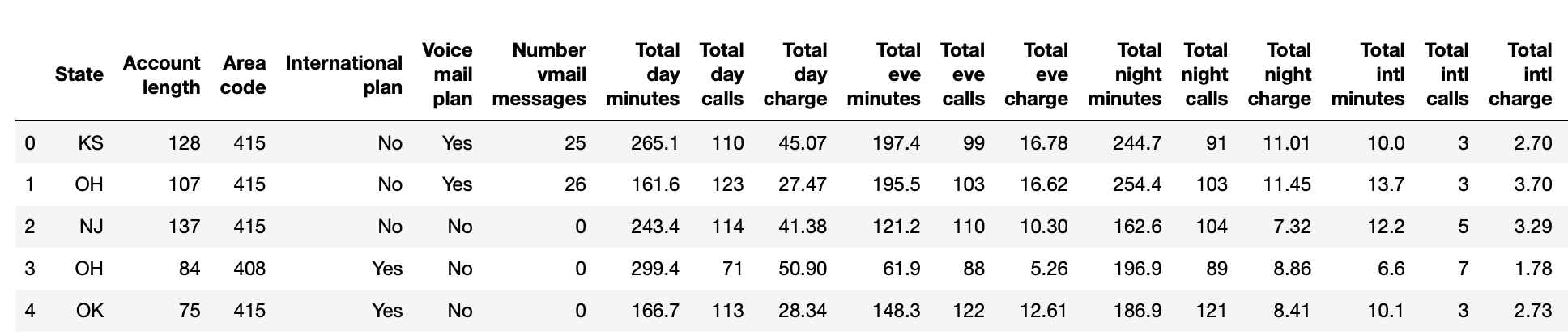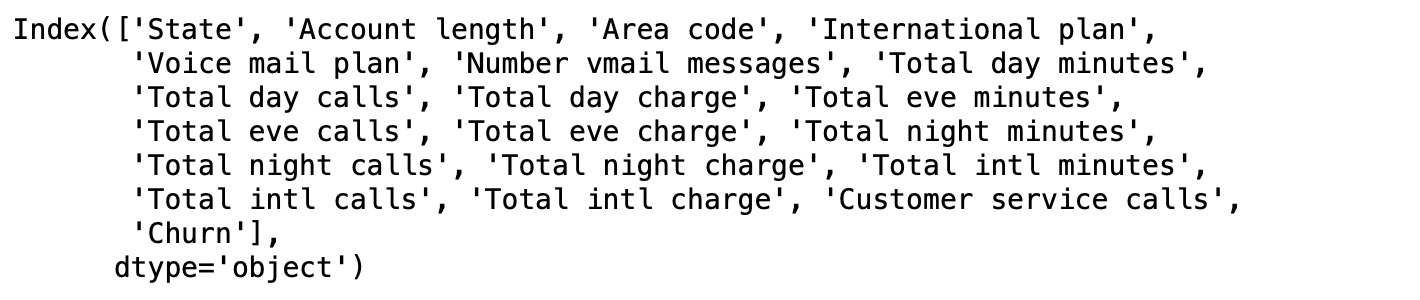Exploratory Data Analysis on Telco Churn Dataset

Code : To find the number of churners and non-churners in the dataset:

 `# Churners vs Non-Churners ` `dataset[``'Churn'``].value_counts() `

Output: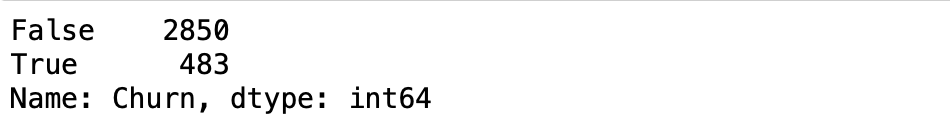Code: To group data by Churn and compute the mean to find out if churners make more customer service calls than non-churners:

 `# Group data by 'Churn' and compute the mean ` `print``(dataset.groupby(``'Churn'``)[``'Customer service calls'``].mean()) `

Output: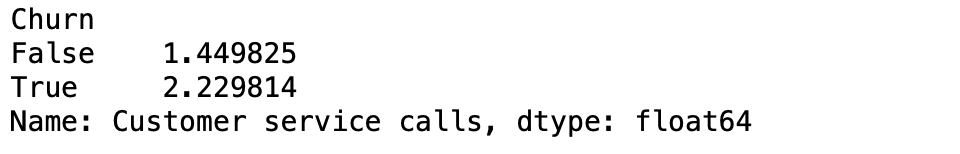Yes! Perhaps unsurprisingly, churners seem to make more customer service calls than non-churners.

Code: To find out if one State has more churners compared to another.

 `# Count the number of churners and non-churners by State ` `print``(dataset.groupby(``'State'``)[``'Churn'``].value_counts()) `

Output: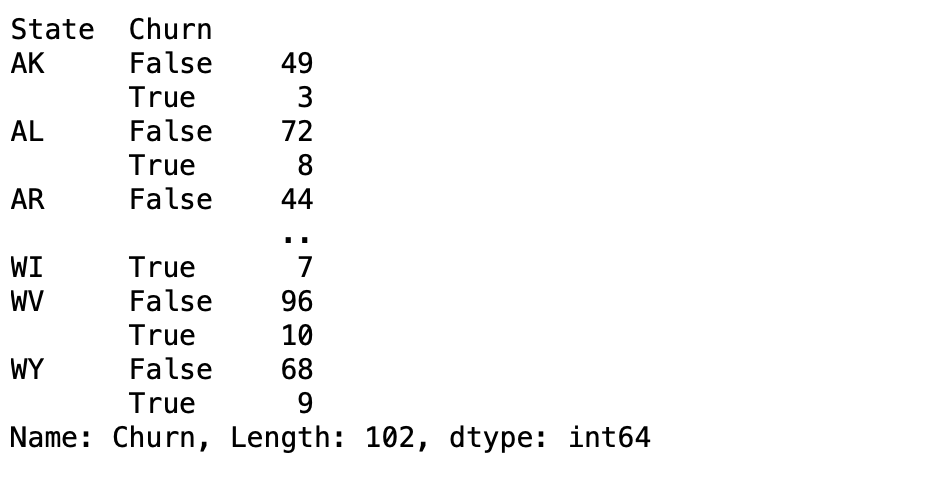While California is the most populous state in the U.S, there are not as many customers from California in our dataset. Arizona (AZ), for example, has 64 customers, 4 of whom ended up churning. In comparison, California has a higher number (and percentage) of customers who churned. This is useful information for a company.

Exploring Data Visualizations : To understand how variables are distributed.

 `# Import matplotlib and seaborn ` `import` `matplotlib.pyplot as plt ` `import` `seaborn as sns ` ` `  `# Visualize the distribution of 'Total day minutes' ` `plt.hist(dataset[``'Total day minutes'``], bins ``=` `100``) ` ` `  `# Display the plot ` `plt.show() `

Output: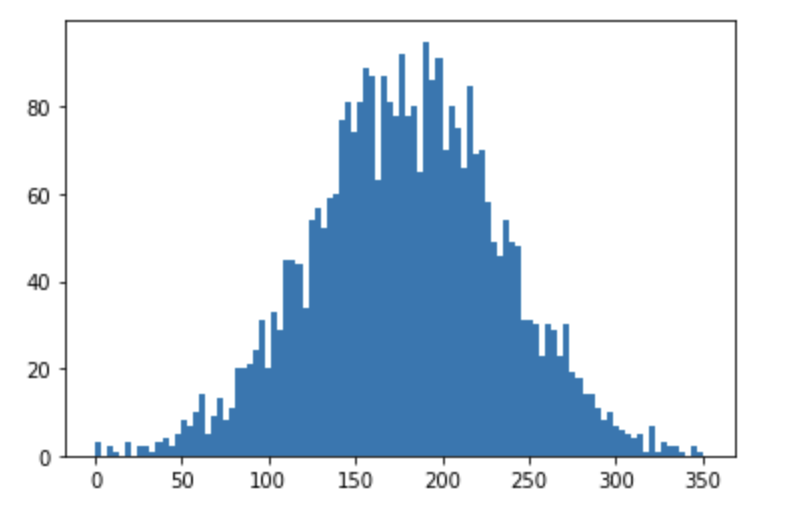Code: To visualize the difference in Customer service calls between churners and non-churners

 `# Create the box plot ` `sns.boxplot(x ``=` `'Churn'``, ` `            ``y ``=` `'Customer service calls'``, ` `            ``data ``=` `dataset, ` `            ``sym ``=` `"",                   ` `            ``hue ``=` `"International plan"``)  ` `# Display the plot ` `plt.show() `

Output: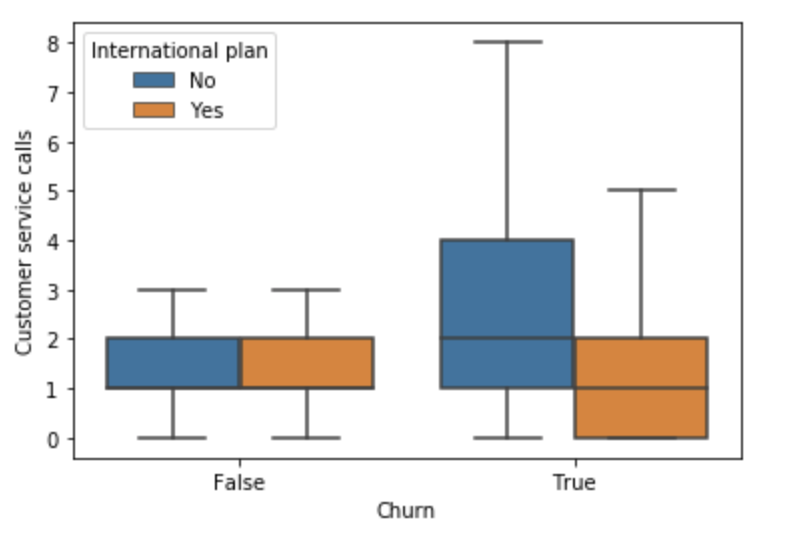It looks like customers who do churn end up leaving more customer service calls unless these customers also have an international plan, in which case they leave fewer customer service calls. This type of information is really useful in better understanding the drivers of churn. It’s now time to learn about how to preprocess your data prior to modelling.

Data Preprocessing for Telco Churn Dataset

Many Machine Learning models make certain assumptions about how the data is distributed. Some of the assumptions are as follows:

• The features are normally distributed
• The features are on the same scale
• The datatypes of features are numeric

In telco churn data, Churn, Voice mail plan, and, International plan, in particular, are binary features that can easily be converted into 0’s and 1’s.

 `# Features and Labels ` `X ``=` `dataset.iloc[:, ``0``:``19``].values ` `y ``=` `dataset.iloc[:, ``19``].values ``# Churn ` ` `  `# Encoding categorical data in X ` `from` `sklearn.preprocessing ``import` `LabelEncoder ` ` `  `labelencoder_X_1 ``=` `LabelEncoder() ` `X[:, ``3``] ``=` `labelencoder_X_1.fit_transform(X[:, ``3``]) ` ` `  `labelencoder_X_2 ``=` `LabelEncoder() ` `X[:, ``4``] ``=` `labelencoder_X_2.fit_transform(X[:, ``4``]) ` ` `  `# Encoding categorical data in y ` `labelencoder_y ``=` `LabelEncoder() ` `y ``=` `labelencoder_y.fit_transform(y) `

Code: Encoding State feature using One hot encoding

 `# Removing extra column to avoid dummy variable trap ` `X_State ``=` `pd.get_dummies(X[:, ``0``], drop_first ``=` `True``) ` ` `  `# Converting X to a dataframe ` `X ``=` `pd.DataFrame(X) ` ` `  `# Dropping the 'State' column ` `X ``=` `X.drop([``0``], axis ``=` `1``) ` ` `  `# Merging two dataframes ` `frames ``=` `[X_State, X] ` `result ``=` `pd.concat(frames, axis ``=` `1``, ignore_index ``=` `True``) ` ` `  `# Final dataset with all numeric features ` `X ``=` `result `

Code : To Create Training and Test sets

 `# Splitting the dataset into the Training and Test sets ` `from` `sklearn.model_selection ``import` `train_test_split ` `X_train, X_test, y_train, y_test ``=` `train_test_split(X, y,  ` `                                                    ``test_size ``=` `0.2``,  ` `                                                    ``random_state ``=` `0``) `

Code: To scale features of the training and test sets

 `# Feature Scaling ` `from` `sklearn.preprocessing ``import` `StandardScaler ` `sc ``=` `StandardScaler() ` `X_train ``=` `sc.fit_transform(X_train) ` `X_test ``=` `sc.transform(X_test) `

Code: To train a Random Forest classifier model on the training set.

 `# Import RandomForestClassifier ` `from` `sklearn.ensemble ``import` `RandomForestClassifier ` ` `  `# Instantiate the classifier ` `clf ``=` `RandomForestClassifier() ` ` `  `# Fit to the training data ` `clf.fit(X_train, y_train) `

Code : Making Predictions

 `# Predict the labels for the test set ` `y_pred ``=` `clf.predict(X_test) `

Code: Evaluating Model Performance

 `# Compute accuracy ` `from` `sklearn.metrics ``import` `accuracy_score ` ` `  `accuracy_score(y_test, y_pred) `

Output:Code : Confusion Matrix

 `from` `sklearn.metrics ``import` `confusion_matrix ` `print``(confusion_matrix(y_test, y_pred)) `

Output:From the confusion matrix, we can compute the following metrics:

• True Positives(TP) = 51
• True Negatives(TN) = 575
• False Positives(FP) = 4
• False Negatives(FN) = 37
• Precision = TP/(TP+FP) = 0.92
• Recall = TP/(TP+FN) = 0.57
• Accuracy = (TP+TN)/(TP+TN+FP+FN) = 0.9385

My Personal Notes arrow_drop_upCheck out this Author's contributed articles.

If you like GeeksforGeeks and would like to contribute, you can also write an article using contribute.geeksforgeeks.org or mail your article to contribute@geeksforgeeks.org. See your article appearing on the GeeksforGeeks main page and help other Geeks.

Please Improve this article if you find anything incorrect by clicking on the "Improve Article" button below.

Article Tags :
Practice Tags :

Be the First to upvote.

Please write to us at contribute@geeksforgeeks.org to report any issue with the above content.